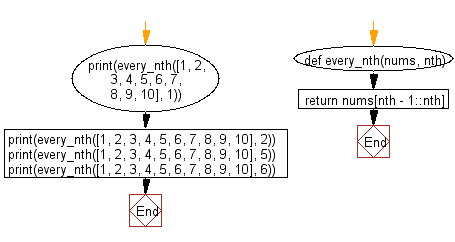﻿ Python: Get every nth element in a given list - w3resource# Python: Get every nth element in a given list

## Python List: Exercise - 269 with Solution

Write a Python program to get the every nth element in a given list.

Use slice notation to create a new list that contains every nth element of the given list

Sample Solution:

Python Code:

``````def every_nth(nums, nth):
return nums[nth - 1::nth]
print(every_nth([1, 2, 3, 4, 5, 6, 7, 8, 9, 10], 1))
print(every_nth([1, 2, 3, 4, 5, 6, 7, 8, 9, 10], 2))
print(every_nth([1, 2, 3, 4, 5, 6, 7, 8, 9, 10], 5))
print(every_nth([1, 2, 3, 4, 5, 6, 7, 8, 9, 10], 6))
```
```

Sample Output:

```[1, 2, 3, 4, 5, 6, 7, 8, 9, 10]
[2, 4, 6, 8, 10]
[5, 10]

```

Flowchart:## Visualize Python code execution:

The following tool visualize what the computer is doing step-by-step as it executes the said program:

Python Code Editor:

Have another way to solve this solution? Contribute your code (and comments) through Disqus.

What is the difficulty level of this exercise?

Test your Python skills with w3resource's quiz

﻿

## Python: Tips of the Day

Floor Division:

When we speak of division we normally mean (/) float division operator, this will give a precise result in float format with decimals.

For a rounded integer result there is (//) floor division operator in Python. Floor division will only give integer results that are round numbers.

```print(1000 // 300)
print(1000 / 300)```

Output:

```3
3.3333333333333335```